# Networks¶

The point and line-segment plotting provided by Datashader can be put together in different ways to visualize specific types of data. For instance, network graph data, i.e., networks of nodes connected by edges, can very naturally be represented by points and lines. Here we will show examples of using Datashader's graph-specific plotting tools, focusing on how to visualize very large graphs while allowing any portion of the rendering pipeline to replaced with components suitable for specific problems.

First, we'll import the packages we are using and demonstrating here.

In :
import math
import numpy as np
import pandas as pd

from datashader.layout import random_layout, circular_layout, forceatlas2_layout

from itertools import chain


## Graph (node) layout¶

Some graph data is inherently spatial, such as connections between geographic locations, and these graphs can simply be plotted by connecting each location with line segments. However, most graphs are more abstract, with nodes having no natural position in space, and so they require a "layout" operation to choose a 2D location for each node before the graph can be visualized. Unfortunately, choosing such locations is an open-ended problem involving a complex set of tradeoffs and complications.

Datashader provides a few tools for doing graph layout, while also working with external layout tools. As a first example, let's generate a random graph, with 100 points normally distributed around the origin and 20000 random connections between them:

In :
np.random.seed(0)
n=100
m=20000

nodes = pd.DataFrame(["node"+str(i) for i in range(n)], columns=['name'])
nodes.tail()

Out:
name
95 node95
96 node96
97 node97
98 node98
99 node99
In :
edges = pd.DataFrame(np.random.randint(0,len(nodes), size=(m, 2)),
columns=['source', 'target'])
edges.tail()

Out:
source target
19995 95 22
19996 16 17
19997 10 17
19998 61 69
19999 56 23

Here you can see that the nodes list is a columnar dataframe with an index value and name for every node. The edges list is a columnar dataframe listing the index of the source and target in the nodes dataframe.

To make this abstract graph plottable, we'll need to choose an x,y location for each node. There are two simple and fast layout algorithms included:

In :
circular  = circular_layout(nodes, uniform=False)
randomloc = random_layout(nodes)
randomloc.tail()

Out:
name x y
95 node95 0.300081 0.003339
96 node96 0.938555 0.354675
97 node97 0.629561 0.575460
98 node98 0.155586 0.970161
99 node99 0.728536 0.435286
In :
cvsopts = dict(plot_height=400, plot_width=400)

def nodesplot(nodes, name=None, canvas=None, cat=None):
canvas = ds.Canvas(**cvsopts) if canvas is None else canvas
aggregator=None if cat is None else ds.count_cat(cat)
agg=canvas.points(nodes,'x','y',aggregator)

tf.Images(nodesplot(randomloc,"Random layout"),
nodesplot(circular, "Circular layout"))

Out:
 Random layout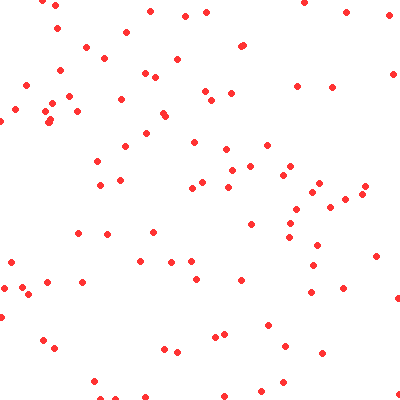Circular layout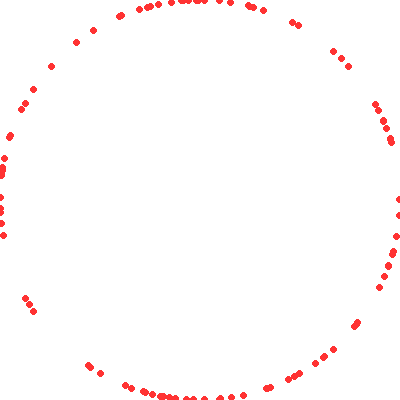The circular layout provides an option to distribute the nodes randomly along the circle or evenly, and here we've chosen the former.

The two layouts above ignore the connectivity structure of the graph, focusing only on the nodes. The ForceAtlas2 algorithm is a more complex approach that treats connections like physical forces (a force-directed approach) in order to construct a layout for the nodes based on the network connectivity:

In :
%time forcedirected = forceatlas2_layout(nodes, edges)
tf.Images(nodesplot(forcedirected, "ForceAtlas2 layout"))

CPU times: user 184 ms, sys: 0 ns, total: 184 ms
Wall time: 188 ms

Out:
 ForceAtlas2 layout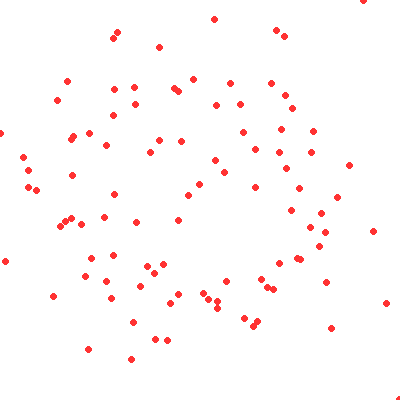This algorithm is designed to place densely connected nodes closer to each other, but of course we will only be able to evaluate how well it has done so once we plot edges (below).

## Edge rendering/bundling¶

Assuming that we have a suitable layout for the nodes, we can now plot the connections between them. There are currently two bundling algorithms provided: drawing a line directly between any connected nodes (connect_edges), and an iterative "bundling" algorithm hammer_bundle (a variant of Hurter, Ersoy, & Telea, ECV-2012) that allows edges to curve and then groups nearby ones together to help convey structure. Rendering direct connections should be very quick, even for large graphs, but bundling can be quite computationally intensive.

In :
def edgesplot(edges, name=None, canvas=None):
canvas = ds.Canvas(**cvsopts) if canvas is None else canvas

def graphplot(nodes, edges, name="", canvas=None, cat=None):
if canvas is None:
xr = nodes.x.min(), nodes.x.max()
yr = nodes.y.min(), nodes.y.max()
canvas = ds.Canvas(x_range=xr, y_range=yr, **cvsopts)

np = nodesplot(nodes, name + " nodes", canvas, cat)
ep = edgesplot(edges, name + " edges", canvas)
return tf.stack(ep, np, how="over", name=name)

In :
cd = circular
fd = forcedirected

%time cd_d = graphplot(cd, connect_edges(cd,edges), "Circular layout")
%time fd_d = graphplot(fd, connect_edges(fd,edges), "Force-directed")
%time cd_b = graphplot(cd, hammer_bundle(cd,edges), "Circular layout, bundled")
%time fd_b = graphplot(fd, hammer_bundle(fd,edges), "Force-directed, bundled")

tf.Images(cd_d,fd_d,cd_b,fd_b).cols(2)

CPU times: user 1.36 s, sys: 3.83 ms, total: 1.37 s
Wall time: 1.37 s
CPU times: user 1.34 s, sys: 3.92 ms, total: 1.34 s
Wall time: 1.34 s
CPU times: user 47.1 s, sys: 124 ms, total: 47.3 s
Wall time: 47.2 s
CPU times: user 36.1 s, sys: 20 ms, total: 36.1 s
Wall time: 36 s

Out:
 Circular layout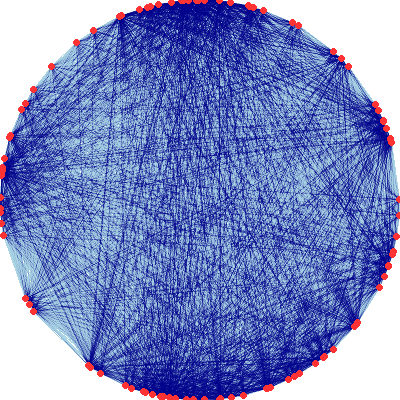Force-directed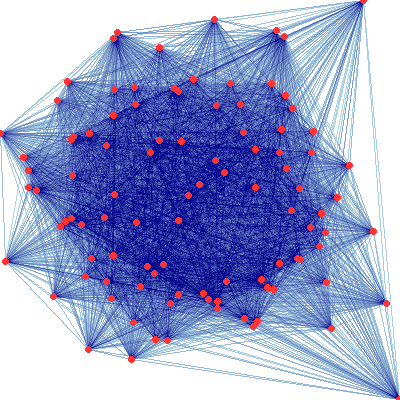Circular layout, bundled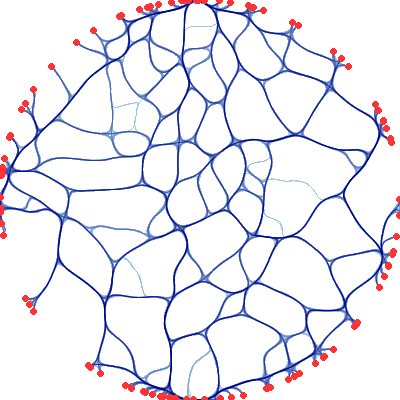Force-directed, bundled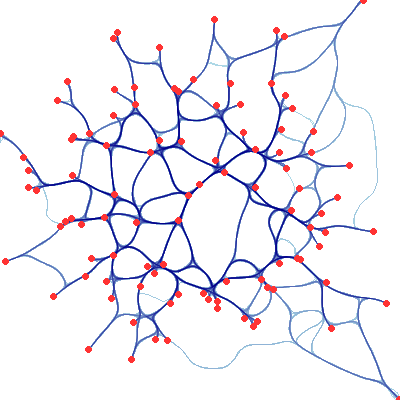The four examples above plot the same network structure by either connecting the nodes directly with lines or bundling the connections, and by using a random layout or a force-directed layout. As you can see, these options have a big effect on the resulting visualization.

Here we'll look more closely at the bundling algorithm, using a simple example where we know the structure: a single node at the center, with random points on a circle around it that connect to the central node (a star graph topology):

In :
n = 75
np.random.seed(0)
x = np.random.random(n)

snodes = pd.DataFrame(np.stack((np.cos(2*math.pi*x),
np.sin(2*math.pi*x))).T, columns=['x','y'])
snodes.iloc = (0.0,0.0)
sedges = pd.DataFrame(list(zip((range(1,n)),*n)),columns=['source', 'target'])
star = snodes,sedges

In :
tf.Images(graphplot(snodes, connect_edges(*star),"Star"),
graphplot(snodes, hammer_bundle(*star),"Star bundled"))

Out:
 Star